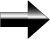Piecewise Functions

Piecewise functions are just like normal functions, except they are defined over a given interval.  Let's look at an example:

{    2x + 1      for   x  ≤  -2

f(x)    =    {         3         for  -2 <  x  ≤   1

{   -3x - 2       for    x   >   1

This function is actually 3 different functions combined.  The key point here is that the inequalities for x tell us which function is on what interval.  In other words, if x is less than or equal to -2, then f(x) = 2x + 1.  If x is between -2 and 1 (including 1, but not -2), then f(x) = 3.  If x is greater than 1, then f(x) = -3x – 2.

Graphing this function is a very good way of illustrating this concept.  There are 3 steps to graphing a piecewise function:

1)  In pencil, sketch a graph of all three functions on the same graph.

2)  Identify the intervals, and erase the graphs of the functions not defined on those intervals.

3)  The last step is to determine if the graph is continuous.  A graph is continuous if you can sketch it without lifting your pencil off the paper. In other words, the graph doesn’t "jump" or have any gaps.  To determine if a function is continuous, we simply evaluate each piece of the function at the end of the intervals.  For our example, we take the 2x + 1 piece, and evaluate it at x = -2:  f(x) = 2x + 1, f(-2) = 2(-2) + 1 = -3.  We can see from the next piece that when -2 < x ≤ 1, f(x) = 3.  Since our last piece left off at -3, we can see that the graph will jump from -3 to 3 right at x  = -2, and therefore, the graph is not continuous.

As you become more comfortable with graphing piecewise functions, you will start to be able to graph them just in one step.  After all, if you can graph a normal function, you can graph a piecewise function!

Main Algebra Page